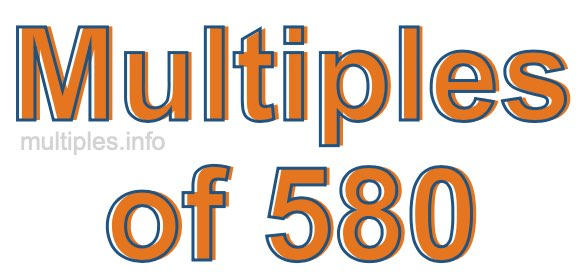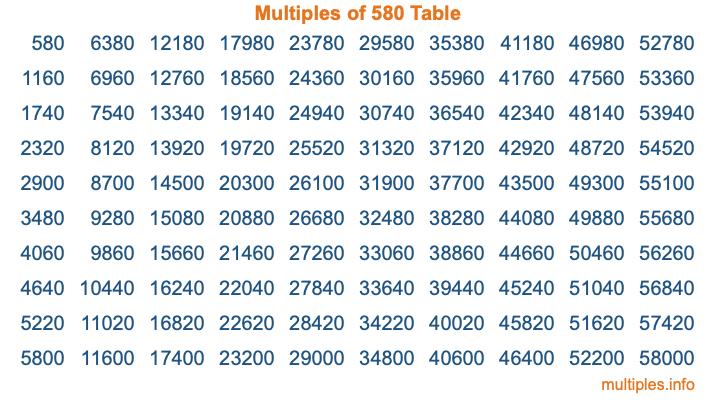Multiples of 580Welcome to the Multiples of 580 page. Here we will first teach you everything you will ever need to know about the multiples of 580, and then give you a study guide summary of everything we taught you to make sure you remember it all. Use this page to look up facts and learn information about the multiples of 580. This page will make you a multiples of five hundred eighty expert!

Definition of Multiples of 580
Multiples of 580 are all the numbers that when divided by 580 equal an integer. Each of the multiples of 580 are called a multiple. A multiple of 580 is created by multiplying 580 by an integer.

Therefore, to create a list of multiples of 580, you start with 1 multiplied by 580, then 2 multiplied by 580, then 3 multiplied by 580, and so on for as long as you want. Thus, the list of the first five multiples of 580 is 580, 1160, 1740, 2320, and 2900. To see a larger list of multiples of 580, see the printable image of Multiples of 580 further down on this page. We also have a category where you can choose any nth multiple of 580.

Multiples of 580 Checker
The Multiples of 580 Checker below checks to see if any number of your choice is a multiple of 580. In other words, it checks to see if there is any number (integer) that when multiplied by 580 will equal your number. To do that, we divide your number by 580. If the the quotient is an integer, then your number is a multiple of 580.

Is  a multiple of 580?

Least Common Multiple of 580 and ...
A Least Common Multiple (LCM) is the lowest multiple that two or more numbers have in common. This is also called the smallest common multiple or lowest common multiple and is useful to know when you are adding our subtracting fractions. Enter one or more numbers below (580 is already entered) to find the LCM.

Check out our LCM Calculator if you need more details about the Least Common Multiple or if you need the LCM for different numbers for adding and subtraction fractions.

nth Multiple of 580
As we stated above, 580 is the first multiple of 580, 1160 is the second multiple of 580, 1740 is the third multiple of 580, and so on. Enter a number below to find the nth multiple of 580.

th multiple of 580

Multiples of 580 vs Factors of 580
580 is a multiple of 580 and a factor of 580, but that is where the similarities end. All postive multiples of 580 are 580 or greater than 580. All positive factors of 580 are 580 or less than 580.

Below is the beginning list of multiples of 580 and the factors of 580 so you can compare:

Multiples of 580: 580, 1160, 1740, 2320, 2900, etc.

Factors of 580: 1, 2, 4, 5, 10, 20, 29, 58, 116, 145, 290, 580

As you can see, the multiples of 580 are all the numbers that you can divide by 580 to get a whole number. The factors of 580, on the other hand, are all the whole numbers that you can multiply by another whole number to get 580.

It's also interesting to note that if a number (x) is a factor of 580, then 580 will also be a multiple of that number (x).

Multiples of 580 vs Divisors of 580
The divisors of 580 are all the integers that 580 can be divided by evenly. Below is a list of the divisors of 580.

Divisors of 580: 1, 2, 4, 5, 10, 20, 29, 58, 116, 145, 290, 580

The interesting thing to note here is that if you take any multiple of 580 and divide it by a divisor of 580, you will see that the quotient is an integer.

Multiples of 580 Table
Below is an image of the first 100 multiples of 580 in a table. The table is in chronological order, column by column. The first column has the first ten multiples of 580, the second column has the next ten multiples of 580, and so on.The Multiples of 580 Table is also referred to as the 580 Times Table or Times Table of 580. You are welcome to print out our table for your studies.

Negative Multiples of 580
Although not often discussed or needed in math, it is worth mentioning that you can make a list of negative multiples of 580 by multiplying 580 by -1, then by -2, then by -3, and so on, to get the following list of negative multiples of 580:

-580, -1160, -1740, -2320, -2900, etc.

Multiples of 580 Summary
Below is a summary of important Multiples of 580 facts that we have discussed on this page. To retain the knowledge on this page, we recommend that you read through the summary and explain to yourself or a study partner why they hold true.

There are an infinite number of multiples of 580.

A multiple of 580 divided by 580 will equal a whole number.

580 divided by a factor of 580 equals a divisor of 580.

The nth multiple of 580 is n times 580.

The largest factor of 580 is equal to the first positive multiple of 580.

580 is a multiple of every factor of 580.

580 is a multiple of 580.

A multiple of 580 divided by a divisor of 580 equals an integer.

580 divided by a divisor of 580 equals a factor of 580.

Any integer times 580 will equal a multiple of 580.

Multiples of a Number
Here you can get the multiples of another number, all with the same attention to detail as we did for multiples of 580 on this page.

Multiples of
Multiples of 581
Did you find our page about multiples of five hundred eighty educational? Do you want more knowledge? Check out the multiples of the next number on our list!